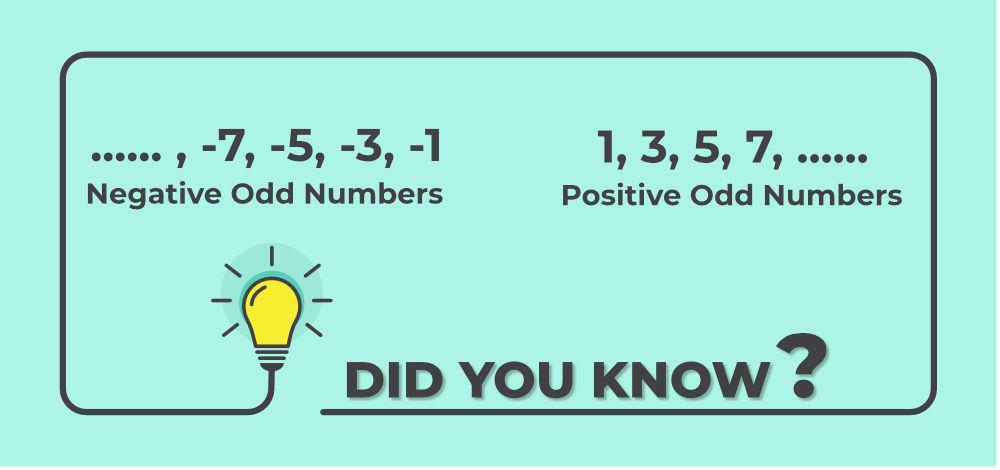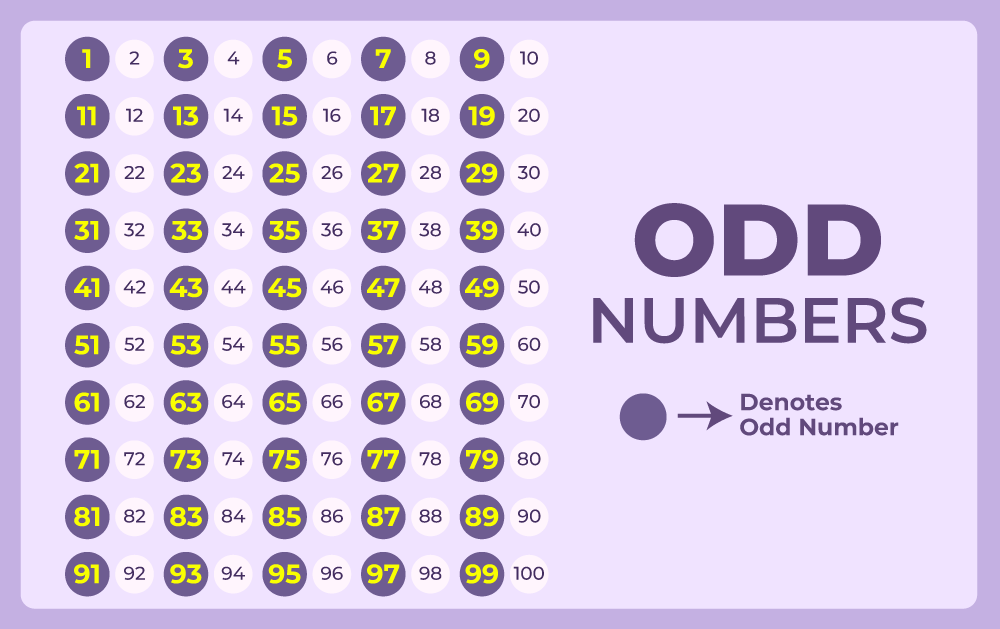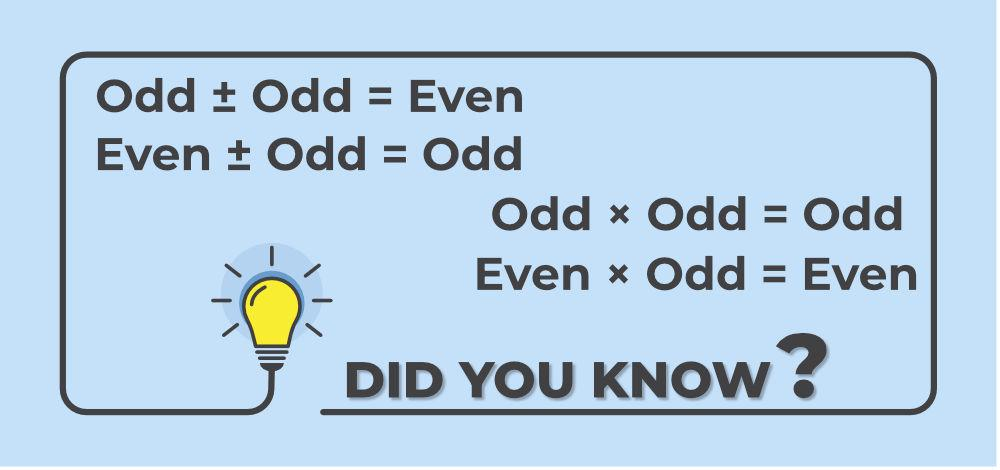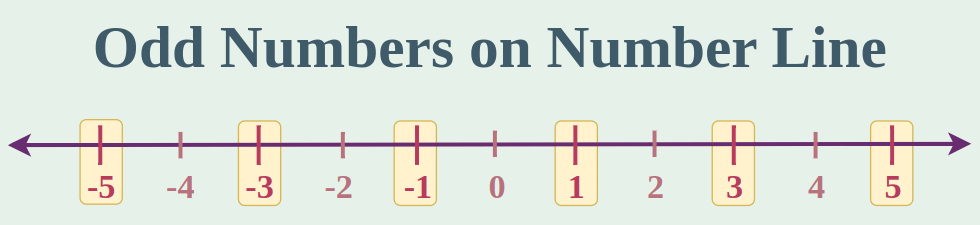# Odd Numbers 1 to 100

Odd numbers 1 to 100 in maths are numbers that are divided by two resulting in the remainder as one. Suppose we are given a number and its division by two results one as the remainder then it is an odd number. For identifying odd numbers the trick is that the one digit of the odd number is always 1, 3, 5, 7, and 9. For example, 91, 45, 77, 3, etc all are odd numbers. Odd numbers can also be negative and their examples are, -61, -13, -27, etc.

Odd Numbers are the complete opposite of even numbers or we can say that odd numbers and even numbers are disjoint sets. In this article, we will learn about – what are odd numbers, list of odd numbers 1 to 100, and examples of odd numbers in detail.

## What are Odd Numbers?

For any number when divided by 2, if the reminder is non zero i.e. 1 then it is an Odd Number. In other words, a number is an Odd number if it is not divisible by 2. For example, 1, 3, 5, 7, 9, etc. One intuitive way to understand Odd numbers is to assume you have n number of fruits, if you try to divide those n fruits among two people such that one person ends up with one more fruit than the other person, then you have an odd number of fruits in the beginning.

### Definition of Odd Numbers

Odd numbers between 1 to 100 are a set of integers (whole numbers) that cannot be exactly divided by 2. In other words, when you divide an odd number by 2, you will always have a remainder of 1.odd numbers

Note: All integers are either even numbers or odd numbers.

## List of Odd Numbers

It is not possible to list out all odd numbers from 1 to 100, as there are an infinite number of them. However, we can list the initial Odd numbers, which include positive Odd numbers such as 1, 3, 5, 7, 9, and so on, extending to infinity, as well as negative odd numbers such as -1, -3, -5, -7, -9, and so on, extending to negative infinity.Positive and negative positive number

## Odd Numbers 1 to 100 Chart

Odd numbers from 1 to 100, can be listed as follows:Odd numbers from 1 to 100

## How to Identify Odd Numbers?

Numbers ending with 1, 3, 5, 7, and 9 are Odd numbers, as only numbers ending with 0, 2, 4, 6, and 8 are divisible by 2. Also if on dividing the number with 2 if the remainder is one then the number is an odd number.

Example: Which of the following is an odd number?

1123, 3214, 12452, 34824, and 98354

Solution:

From the given number 1123 is an odd number because, on dividing with 2 it gives the remainder as 1.

## Even and Odd Numbers

There are some differences between even and odd numbers, as follows:

Even Numbers Odd Numbers
Numbers divisible exactly by 2 are even numbers. When divided by 2, those numbers give 1 as a reminder and are known as Odd numbers.
Some examples, of even numbers, are 2, 4, 6, 8,10, etc. Some examples of odd numbers are 1, 3, 5, 7, 9, etc.
Even numbers can be represented by 2k, where all k belongs to integers. Odd numbers can be represented by 2k+1, where all k belongs to integers.

## Sum of Odd Numbers from 1 to 100

The sum of all odd numbers from 1 to 100 can be calculated using the formula S = n/2(first odd number + last odd number), where n is the total count of odd numbers within the range. As there are 50 odd numbers (n = 50) between 1 and 100, we can substitute these values into the formula:This simplifies to:Resulting in:

S = 2500

Therefore, the sum of all odd numbers from 1 to 100 is 2500.

## Even and Odd Numbers 1 to 100

There are 50 even numbers and 50 odd numbers between 1 and 100. The list of even numbers is: 2, 4, 6, 8, 10, 12, 14, 16, 18, 20, 22, 24, 26, 28, 30, 32, 34, 36, 38, 40, 42, 44, 46, 48, 50, 52, 54, 56, 58, 60, 62, 64, 66, 68, 70, 72, 74, 76, 78, 80, 82, 84, 86, 88, 90, 92, 94, 96, 98, 100.

Similarly, the list of odd numbers is: 1, 3, 5, 7, 9, 11, 13, 15, 17, 19, 21, 23, 25, 27, 29, 31, 33, 35, 37, 39, 41, 43, 45, 47, 49, 51, 53, 55, 57, 59, 61, 63, 65, 67, 69, 71, 73, 75, 77, 79, 81, 83, 85, 87, 89, 91, 93, 95, 97, 99.

## Odd Prime Numbers from 1 to 100

We define prime numbers as those having only two factors, 1 and the number itself, while odd numbers are not divisible by 2. It’s worth noting that certain odd numbers, such as 9, 15, 21, 25, and others, are not prime. Additionally, 2 is a prime number but not odd.

To compile a list of odd prime numbers from 1 to 100, we can present it as follows: 3, 5, 7, 11, 13, 17, 19, 23, 29, 31, 37, 41, 43, 47, 53, 59, 61, 67, 71, 73, 79, 83, 89, and 97. In total, there are 24 odd prime numbers within this range.

## Properties of Odd Numbers

All the Odd numbers can be represented as 2k + 1, where all k belongs to integers. For example, 13 can be written as 2 × 6 + 1, -11 can be written as 2 × (-6) + 1, and 21 can be written as 2 × 10 + 1, etc.

There are various property of odd numbers explained in the table below,

Property

Operation

Example

Odd + Odd = Even

3 + 7 = 10

Property of Subtraction

Odd – Odd = Even

7 – 3 = 4

Property of Multiplication

Odd × Odd = Even

3 × 7 = 21

All these properties are explained in detail below:

• Addition of two Odd Numbers results in an Even Number.
• For example, 1+3=4, 5+11=16, and -3+5=2 etc.
• Addition of one Odd and one Even number results in an Odd Number.
• For example, 2+3=5, -1,4=3, and 11+4=15 etc.

### Property of Subtraction

• Subtraction between two Odd numbers always results in an even number.
• For example, 3-5=-2, 7-1=6, and -5-3=-8 etc.
• Subtraction between one Odd and one Even number always results in an Odd number.
• For example, 1-4=-3, -1-2=-3, and 2-5=-3, etc.

### Property of Multiplication

• Multiplication of two Odd numbers always results in an Odd number.
• For example, 3×5=15, 1×17=17, and 13×5=65 etc.
• Multiplication of one odd and one even number always results in an Even number.
• For example, 4×5=20, 2×13=26, and 11×4=44 etc.Properties of odd numbers

## Types of Odd Numbers

Various types of Odd Numbers are as follows,

• Consecutive Odd Numbers
• Composite Odd Numbers
• Prime Odd Numbers

Now let’s learn about them in detail.

### Consecutive Odd Numbers

For any number to be consecutive, they need to follow each other in order, and if numbers are consecutive as well as Odd in nature then those are called consecutive odd numbers. Examples of consecutive odd numbers include 1, 3, 5, 7, and 9 (the first five consecutive odd natural numbers), and 11, 13, 15, 17, and 19. If we have an odd number a, we can determine the next consecutive odd number by adding 2 to it, i.e., a+2. It is important to note that the difference between any two consecutive odd or even numbers is always 2.

### Composite Odd Numbers

Positive integers that have factors other than 1 and themselves are called composite numbers. For a number to be considered a composite odd number, a number must be both odd and composite. For instance, 9 is a composite odd number because it is divisible by 3, and when divided by 2, it gives a remainder of 1. Other examples of composite odd numbers include 15, 27, 35, 65, and so on.

### Prime Odd Numbers

Except for the number 2, all prime numbers are odd. This is because, except for 2, all even numbers have 2 as a factor, making them composite numbers. However, not all odd numbers are prime since the product of two odd numbers is also an odd number, but it cannot be a prime number because it has two factors. A prime number is defined as an odd number that has no factors other than 1 and itself.

Some examples of prime as well as odd numbers are 3, 5, 7, 11, 13, 17, 19, etc.

Note: All Prime Numbers are Odd Numbers except for 2 which is Even Number

### Smallest Odd Number

The smallest odd number is 1 as is the smallest number in the lot of odd number. Other odd number are, 1, 3, 5, 7, 9,…

### First 10 Odd Numbers

The first 10 odd numbers are,

1, 3, 5, 7, 9, 11, 13, 15, 17, and 19.

### Odd Prime Numbers

All the prime number except for 2 are odd numbers because all even numbers have at least one factor that is 2. Various odd prime numbers are,

1, 3, 5, 7, 11, 13, 17, 19, 23, 29, etc.

### Odd Natural Numbers

Natural Numbers are the number that are used for counting numbers. Various odd natural number are,

1, 3, 5, 7, 9,…

## What is Smallest Odd Composite Number?

The smallest odd composite number is 9, as the list of first odd natural numbers is 1, 3, 5, 7, 9, 11, 13,… and the first composite number is 4 which is not an odd number, a second composite number is 6 which is also not an odd number as it is divisible by 2, third composite number is 8 which is again an even number. So, the fourth composite number which is 9 is the first odd composite number. Thus, 9 is the smallest odd composite number.

## Odd Number on Number Line

A number line is a line in which numbers are marked and is used to marks the position of various numbers and perform all sort of mathematical operations such as addition, subtraction, and others.

Odd numbers are easily represented on the number line. They are represented by skipping one number and marking the other number starting from any odd number.

The image added below shows odd numbers on a number line,## Solved Examples of Odd Numbers 1 to 100

Example 1: How many odd numbers are between 1 and 150 (including 1 and 150)?

Solution:

Every other number is an odd number thus half of the all the numbers are odd.

So, between 1 and 150 (including 1 and 150), there are 150 numbers,

Thus, half of 150 number are odd.

There are 75 odd numbers between 1 and 150.

Example 2: Find the units digit of 3201.

Solution:

The units digit of any power of 3 is cyclical and follows a pattern. The pattern for 3 is 3, 9, 7, 1.

Therefore, the units digit of 3201 is the same as the units digit of 3x where x is the remainder when 201 is divided by 4.

and the remainder when 201 is divided by 4 is 1, so the units digit of 3201 is the same as the units digit of 31, which is 3.

Therefore, the unit digit of 3201 is 3.

Example 3: Find the product of all odd numbers between 1 to 9.

Solution:

Odd numbers between 1 to 9 are 1, 3, 5, 7, 9.

Product of all odd numbers between 1 to 9 are

= 1 × 3 × 5 × 7 × 9

= 945

Example 4: Determine whether the following numbers are even or odd,

• 73
• 2 + 4 + 6 + 8
• 99 – 67

Solution:

73 is not divisible by 2, so it is an odd number

Sum of first four even numbers is 2 + 4 + 6 + 8 = 20. Since 20 is divisible by 2, it is not an odd number, so it is an even number

99 – 67 = 32. Since 32 is divisible by 2, it is not an odd number, so it is an even number

Thus, only 73 is Odd Number

Example 5: Find the sum odd numbers from 10 to 20.

Solution:

Odd Numbers from 10 to 20 are 11, 13, 15, 17

Sum = 11 + 13 + 15 + 17

Sum = 56

Thus, the sum of odd numbers from 10 to 20 is 56.

Example 6: Find the difference of 27 and 13

Solution:

Difference of 27 and 13

= 27 – 13

= 14

## Practice Questions on Odd Numbers 1 to 100

Q1. Find the sum of odd numbers from 20 to 40

Q2. Check wether they are odd numbers or not, 78, 23, 46, 91.

Q3. Find the product of 13 and 21.

Q4. How many odd numbers are from 50 to 100?

## FAQs on Odd Numbers 1 to 100

### 1. What are Odd Numbers in Maths?

Those numbers which are exactly not divisible by 2, are called Odd numbers. For example, 3, 5, 7, 15, etc.

### 2. What is the HCF of two consecutive odd numbers?

Consecutive number are those number which follow each other in order. Thus, list consecutive of Odd natural number is 1, 3, 5, 7, 9, 11, 13, . . .

• For 1 and 3, only common factor is 1.
• For 3 and 5, only common factor is 1.
• For 5 and 7, only common factor is 1…

Similarly for each consecutive pair only possible common factor is 1.

So, HCF of two conscutive odd numbers is 1.

### 3. How Many Odd Numbers are Between 1 and 100?

As every other integer is odd and between 1 and 100 there are 98 number(not including 1 and 100). Thus, half of them must be even and half of them are odd number. Therefore there are 49 Odd numbers between 1 and 100.

### 4. What is Sum of First ‘n’ Odd Natural Numbers?

1 + 3 + 5 + 7 +… up to n terms

Consider this Arithmetic Progression, a = 1, d = 2 and using summation of n terms of A.P.

Required Sum = n/2{2a + (n – 1)d}

= n/2{2 + (n -1)2}

= n/2{2 + 2n – 2}

= n/2{2n} = n2

Thus, sum of first n odd natural numbers is n2.

### 5. Is Zero an Odd Number?

No, zero is not an odd number, because it is not divisible by 2.

### 6. What is General Form of Even Numbers?

The general form of an odd number is 2n – 1, where n is any integer.

### 7. What Odd Numbers are Prime?

Various odd prime numbers are,

1, 3, 5, 7, 9, 11, …

### 8. What is Average of Odd Numbers 1 to 100?

The average of odd numbers 1 to 100 is 50.

### 9. How many Odd Numbers are there from 1 to 100?

There are 50 odd numbers from 1 to 100 and these are 1, 3, 5, 7, 9, 11, 13, 15, 17, 19, 21, 23, 25, 27, 29, 31, 33, 35, 37, 39, 41, 43, 45, 47, 49, 51, 53, 55, 57, 59, 61, 63, 65, 67, 69, 71, 73, 75, 77, 79, 81, 83, 85, 87, 89, 91, 93, 95, 97, 99.

Whether you're preparing for your first job interview or aiming to upskill in this ever-evolving tech landscape, GeeksforGeeks Courses are your key to success. We provide top-quality content at affordable prices, all geared towards accelerating your growth in a time-bound manner. Join the millions we've already empowered, and we're here to do the same for you. Don't miss out - check it out now!

Previous
Next